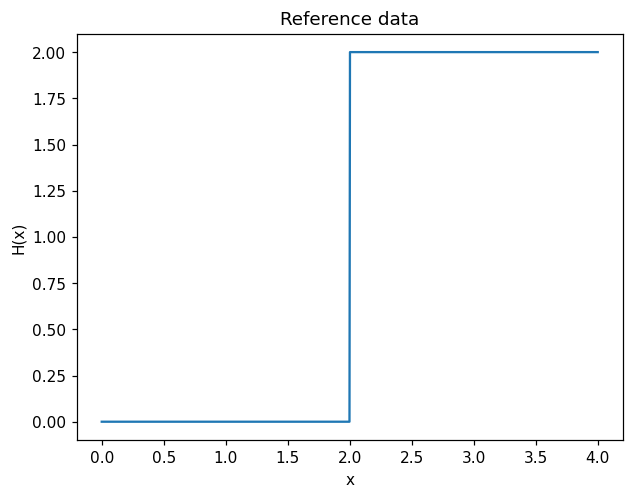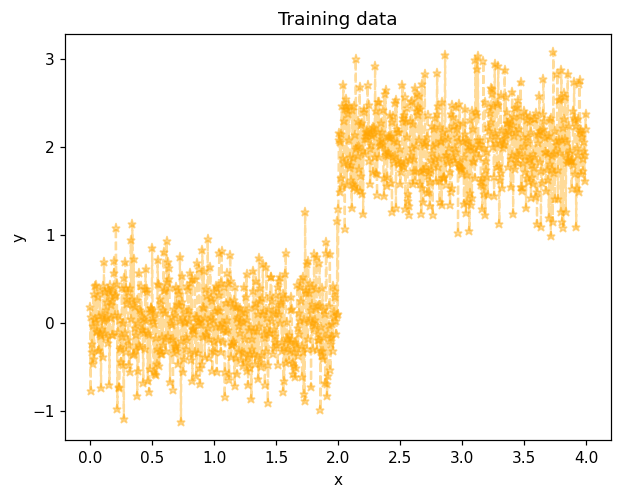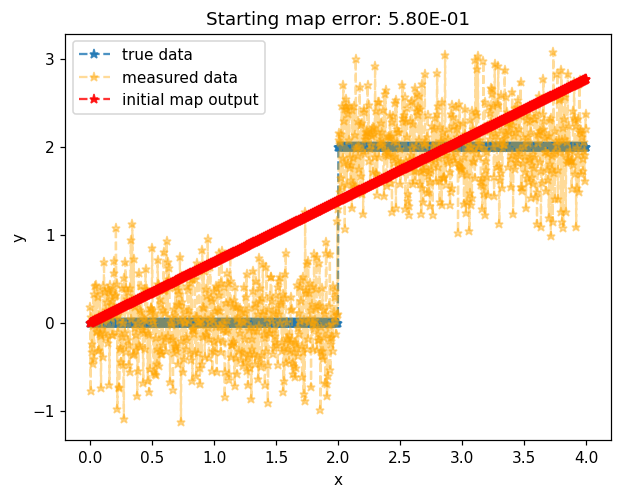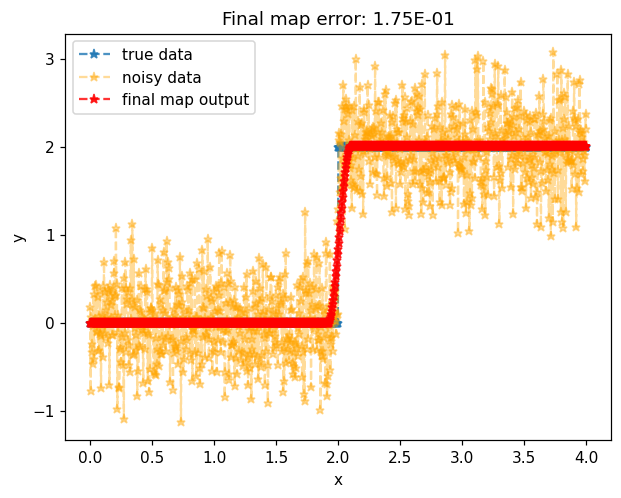Launch this notebook on on mybinder.org:# Monotone least squares#

The objective of this example is to show how to build a transport map to solve monotone regression problems using MParT.

## Problem formulation#

One direct use of the monotonicity property given by the transport map approximation to model monotone functions from noisy data. This is called isotonic regression and can be solved in our setting by minimizing the least squares objective function

$J(\mathbf{w})= \frac{1}{2} \sum_{i=1}^N \left(S(x^i;\mathbf{w}) - y^i \right)^2,$

where $$S$$ is a monotone 1D map with parameters (polynomial coefficients) $$\mathbf{w}$$ and $$y^i$$ are noisy observations. To solve for the map parameters that minimize this objective we will use a gradient-based optimizer. We therefore need the gradient of the objective with respect to the map paramters. This is given by

$\nabla_\mathbf{w} J(\mathbf{w})= \sum_{i=1}^N \left(S(x^i;\mathbf{w}) - y^i \right)^T\left[\nabla_\mathbf{w}S(x^i;\mathbf{w})\right]$

The implementation of S(x) we’re using from MParT, provides tools for both evaluating the map to compute $$S(x^i;\mathbf{w})$$ but also evaluating computing the action of $$\left[\nabla_\mathbf{w}S(x^i;\mathbf{w})\right]^T$$ on a vector, which is useful for computing the gradient. Below, these features are leveraged when defining an objective function that we then minimize with the BFGS optimizer implemented in scipy.minimize.

## Imports#

First, import MParT and other packages used in this notebook. Note that it is possible to specify the number of threads used by MParT by setting the KOKKOS_NUM_THREADS environment variable before importing MParT.

:

import numpy as np
from scipy.optimize import minimize
import matplotlib.pyplot as plt
import os

import mpart as mt
plt.rcParams['figure.dpi'] = 110

Kokkos is usingKokkos::OpenMP::initialize WARNING: You are likely oversubscribing your CPU cores.

Kokkos::OpenMP::initialize WARNING: You are likely oversubscribing your CPU cores.
Detected: 2 cores per node.
Detected: 1 MPI_ranks per node.


## Generate training data#

### True model#

Here we choose to use the step function $$H(x)=\text{sgn}(x-2)+1$$ as the reference monotone function. It is worth noting that this function is not strictly monotone and is only piecewise continuous.

:

# variation interval
num_points = 1000
xmin, xmax = 0, 4
x = np.linspace(xmin, xmax, num_points)[None,:]

y_true = 2*(x>2)

plt.figure()
plt.title('Reference data')
plt.plot(x.flatten(),y_true.flatten())
plt.xlabel('x')
plt.ylabel('H(x)')
plt.show()### Training data#

Training data $$y^i$$ in the objective defined above are simulated by pertubating the reference data with a white Gaussian noise with a $$0.4$$ standard deviation.

:

noisesd = 0.4

y_noise = noisesd*np.random.randn(num_points)
y_measured = y_true + y_noise

plt.figure()
plt.title('Training data')
plt.plot(x.flatten(),y_measured.flatten(),color='orange',marker='*',linestyle='--',label='measured data', alpha=0.4)
plt.xlabel('x')
plt.ylabel('y')
plt.show()## Map initialization#

We use the previously generated data to train the 1D transport map. In 1D, the map complexity can be set via the list of multi-indices. Here, map complexity can be tuned by setting the max_order variable.

### Multi-index set#

:

# Define multi-index set
max_order = 5
multis = np.linspace(0,max_order,6).reshape(-1,1).astype(int)
mset = mt.MultiIndexSet(multis)
fixed_mset = mset.fix(True)

# Set options and create map object
opts = mt.MapOptions()

monotone_map = mt.CreateComponent(fixed_mset, opts)


### Plot initial approximation#

:

# Before optimization
map_of_x_before = monotone_map.Evaluate(x)
error_before = np.sum((map_of_x_before - y_measured)**2)/x.shape

# Plot data and initial approximation
plt.figure()
plt.title('Starting map error: {:.2E}'.format(error_before))
plt.plot(x.flatten(),y_true.flatten(),'*--',label='true data', alpha=0.8)
plt.plot(x.flatten(),y_measured.flatten(),'*--',label='measured data',color='orange', alpha=0.4)
plt.plot(x.flatten(),map_of_x_before.flatten(),'*--',label='initial map output', color="red", alpha=0.8)
plt.xlabel('x')
plt.ylabel('y')
plt.legend()
plt.show()Initial map with coefficients set to zero result in the identity map.

## Transport map training#

### Objective function#

:

# Least squares objective
def objective(coeffs, monotone_map, x, y_measured):
monotone_map.SetCoeffs(coeffs)
map_of_x = monotone_map.Evaluate(x)
return np.sum((map_of_x - y_measured)**2)/x.shape

monotone_map.SetCoeffs(coeffs)
map_of_x = monotone_map.Evaluate(x)


#### Optimization#

:

# Optimize
optimizer_options={'gtol': 1e-3, 'disp': True}
res = minimize(objective, monotone_map.CoeffMap(), args=(monotone_map, x, y_measured), jac=grad_objective, method='BFGS', options=optimizer_options)

# After optimization
map_of_x_after = monotone_map.Evaluate(x)
error_after = objective(monotone_map.CoeffMap(), monotone_map, x, y_measured)

Optimization terminated successfully.
Current function value: 0.168862
Iterations: 53
Function evaluations: 56


### Plot final approximation#

:

plt.figure()
plt.title('Final map error: {:.2E}'.format(error_after))
plt.plot(x.flatten(),y_true.flatten(),'*--',label='true data', alpha=0.8)
plt.plot(x.flatten(),y_measured.flatten(),'*--',label='noisy data', color='orange',alpha=0.4)
plt.plot(x.flatten(),map_of_x_after.flatten(),'*--',label='final map output', color="red", alpha=0.8)
plt.xlabel('x')
plt.ylabel('y')
plt.legend()
plt.show()Unlike the true underlying model, map approximation gives a strict coninuous monotone regression of the noisy data.